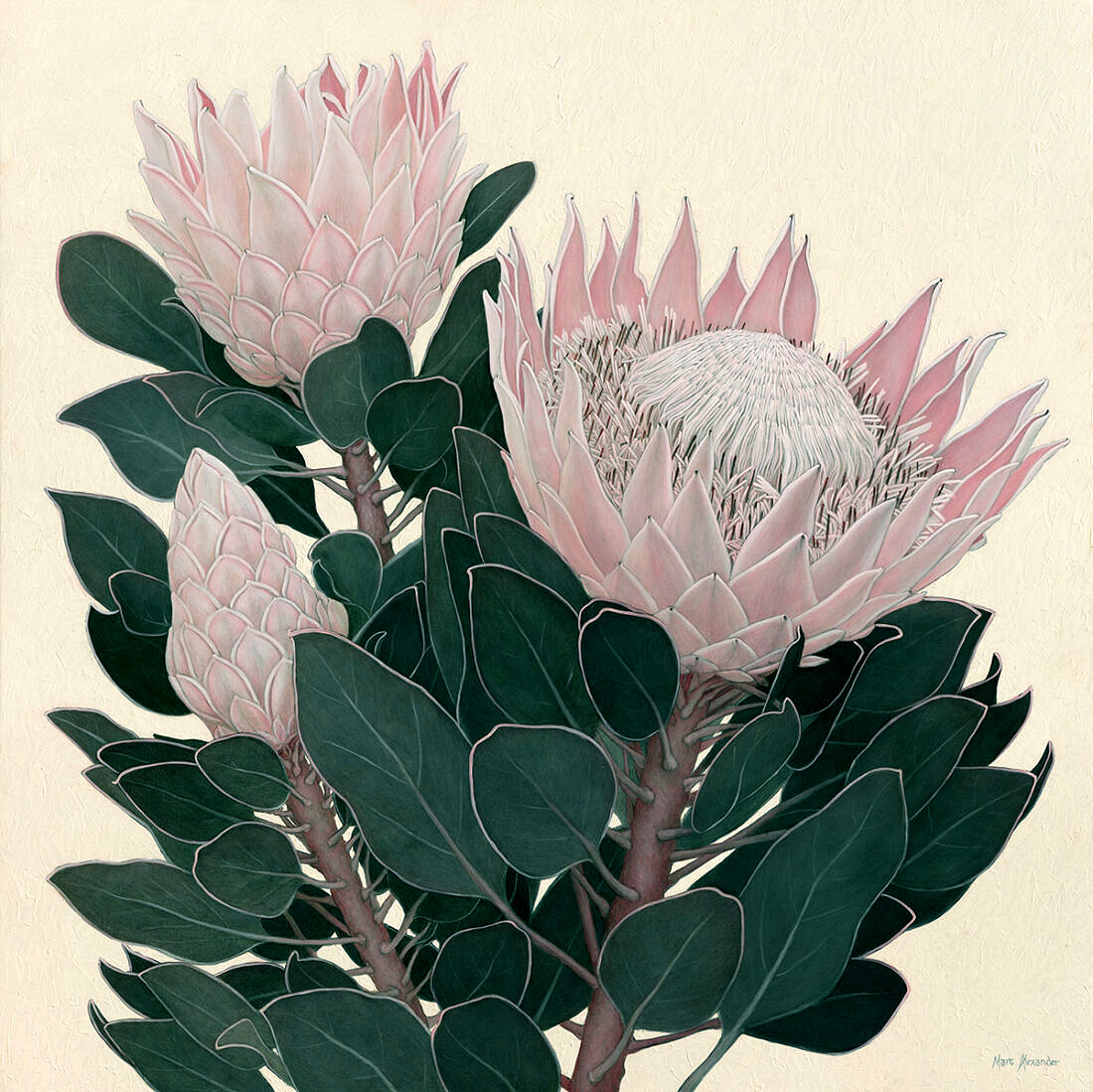##### King Protea I
Currency conversion from USD to: [convert number=2300.00 from="usd" to="zar"] ZAR | [convert number=2300.00 from="usd" to="gbp"] GBP | [convert number=2300.00 from="usd" to="eur"] EUR     Medium: Oil on Canvas Size: 100cm by 100cm SOLD
\$ 2,300.00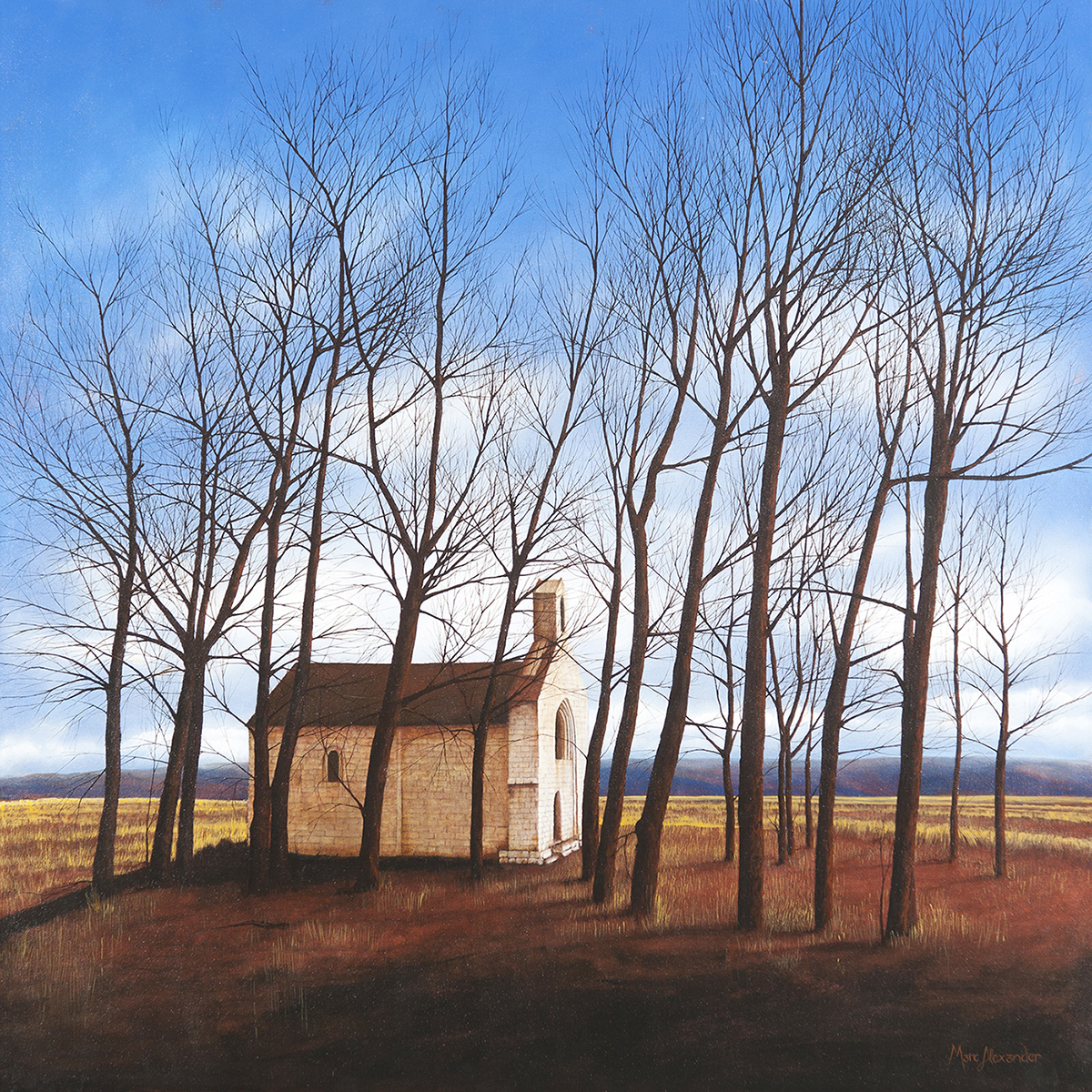##### Winter
Currency conversion from USD to: [convert number=2500.00 from="usd" to="zar"] ZAR | [convert number=2500.00 from="usd" to="gbp"] GBP | [convert number=2500.00 from="usd" to="eur"] EUR     Medium: Oil on Canvas Size: 100cm by 100cm SOLD
\$ 2,500.00##### Spring
Currency conversion from USD to: [convert number=2500.00 from="usd" to="zar"] ZAR | [convert number=2500.00 from="usd" to="gbp"] GBP | [convert number=2500.00 from="usd" to="eur"] EUR     Medium: Oil on Canvas Size: 100cm by 100cm SOLD
\$ 2,500.00##### Enchanted Forest II
Currency conversion from USD to: [convert number=2500.00 from="usd" to="zar"] ZAR | [convert number=2500.00 from="usd" to="gbp"] GBP | [convert number=2500.00 from="usd" to="eur"] EUR     Medium: Oil on Canvas Size: 100cm by 100cm SOLD
\$ 2,500.00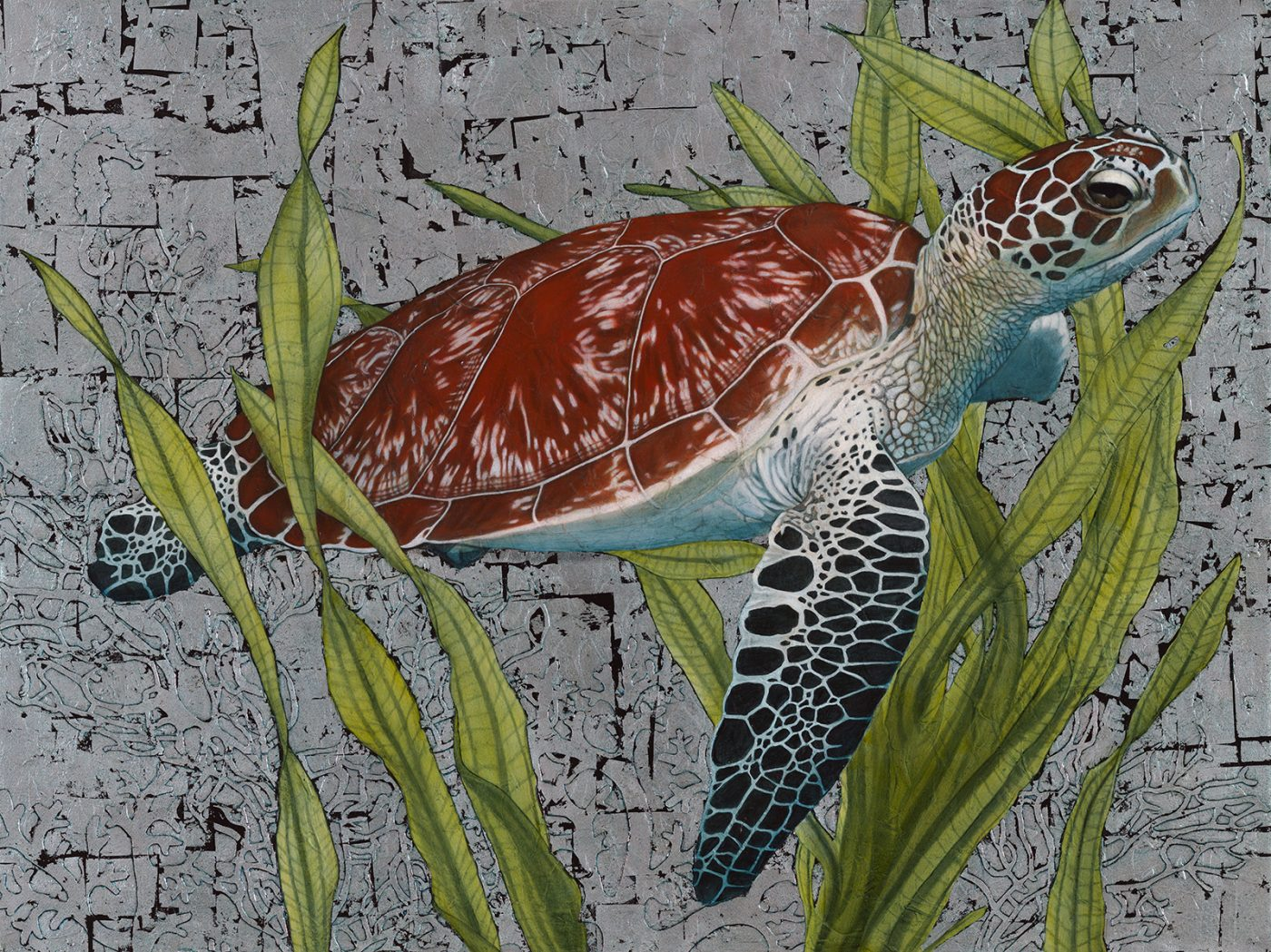##### Hawksbill Sea Turtle
Currency conversion from USD to: [convert number=2550.00 from="usd" to="zar"] ZAR | [convert number=2550.00 from="usd" to="gbp"] GBP | [convert number=2550.00 from="usd" to="eur"] EUR   Medium: Oil and Silver Leaf on Canvas Size: 90cm by 120cm SOLD
\$ 2,550.00##### African Buffalo
Currency conversion from USD to: [convert number=3450.00 from="usd" to="zar"] ZAR | [convert number=3450.00 from="usd" to="gbp"] GBP | [convert number=3450.00 from="usd" to="eur"] EUR     Medium: Oil and Gold Leaf on Canvas Size: 90cm by 120cm SOLD
\$ 3,450.00##### Bateleur Eagle I
Currency conversion from USD to: [convert number=3450.00 from="usd" to="zar"] ZAR | [convert number=3450.00 from="usd" to="gbp"] GBP | [convert number=3450.00 from="usd" to="eur"] EUR     Medium: Oil and Gold Leaf on Canvas Size: 90cm by 120cm SOLD
\$ 3,450.00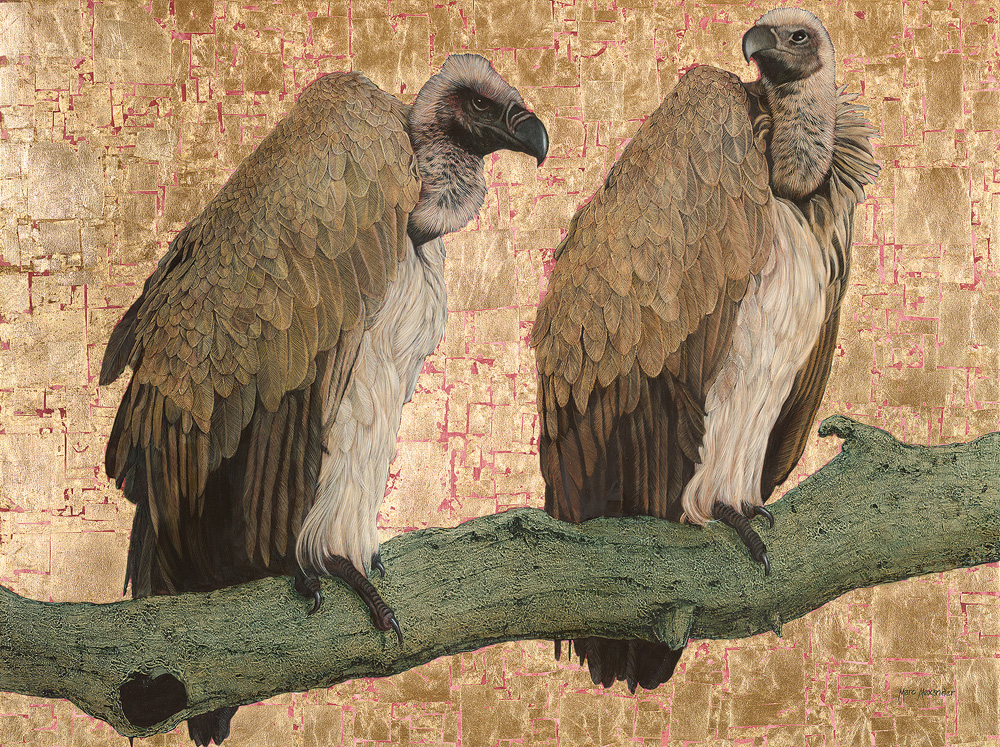##### Cape Vultures
Currency conversion from USD to: [convert number=3650.00 from="usd" to="zar"] ZAR | [convert number=3650.00 from="usd" to="gbp"] GBP | [convert number=3650.00 from="usd" to="eur"] EUR     Medium: Oil and Gold Leaf on Canvas Size: 90cm by 120cm SOLD
\$ 3,650.00##### Rhino II
Currency conversion from USD to: [convert number=3650.00 from="usd" to="zar"] ZAR | [convert number=3650.00 from="usd" to="gbp"] GBP | [convert number=3650.00 from="usd" to="eur"] EUR     Medium: Oil and Gold Leaf on Canvas Size: 90cm by 120cm SOLD
\$ 3,650.00##### Mighty Tusker
Currency conversion from USD to: [convert number=3650.00 from="usd" to="zar"] ZAR | [convert number=3650.00 from="usd" to="gbp"] GBP | [convert number=3650.00 from="usd" to="eur"] EUR     Medium: Oil and Gold Leaf on Canvas Size: 90cm by 120cm SOLD
\$ 3,650.00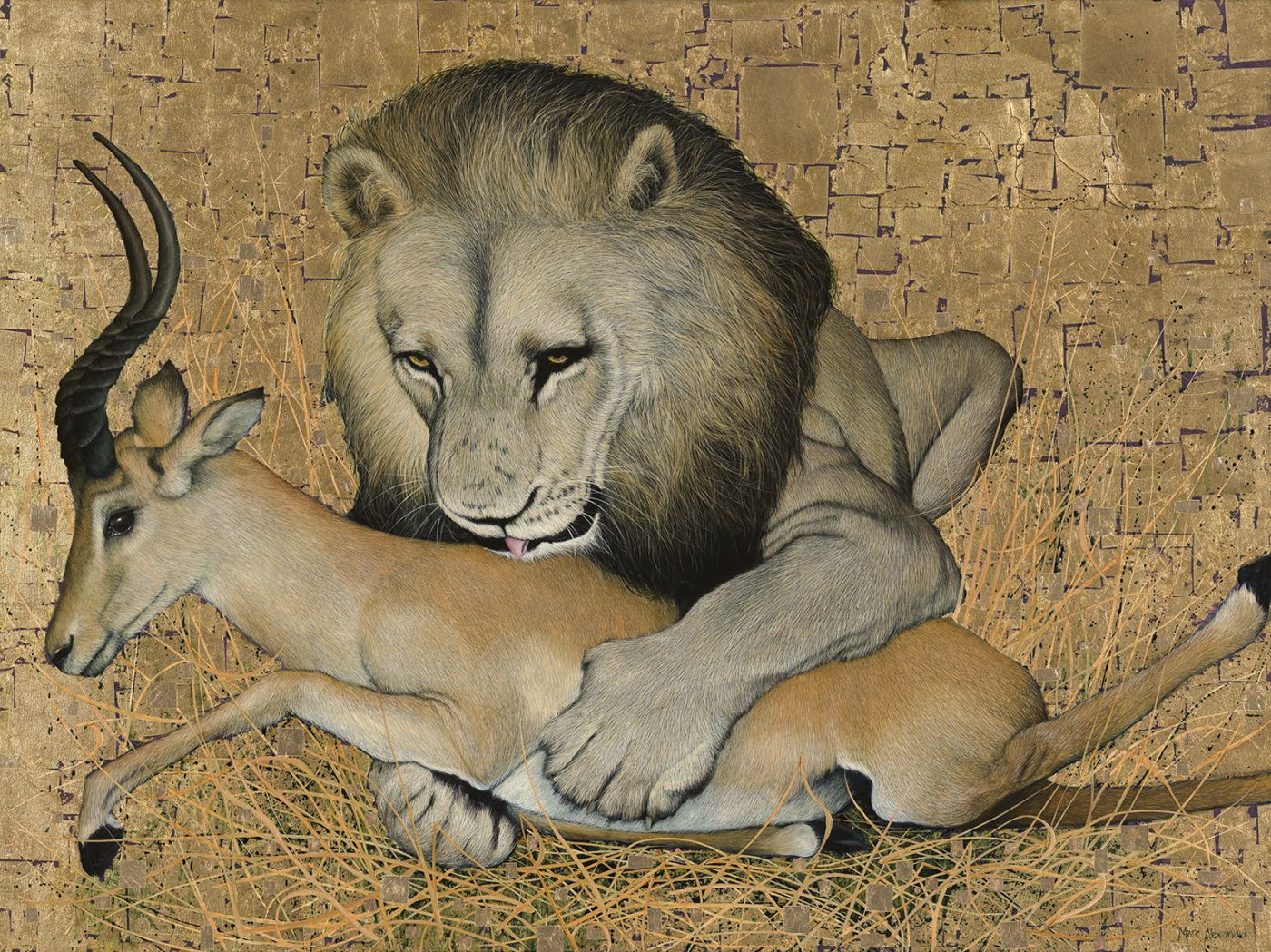##### The Kill
Currency conversion from USD to: [convert number=3650.00 from="usd" to="zar"] ZAR | [convert number=3650.00 from="usd" to="gbp"] GBP | [convert number=3650.00 from="usd" to="eur"] EUR     Medium: Oil and Gold Leaf on Canvas Size: 90cm by 120cm SOLD
\$ 3,650.00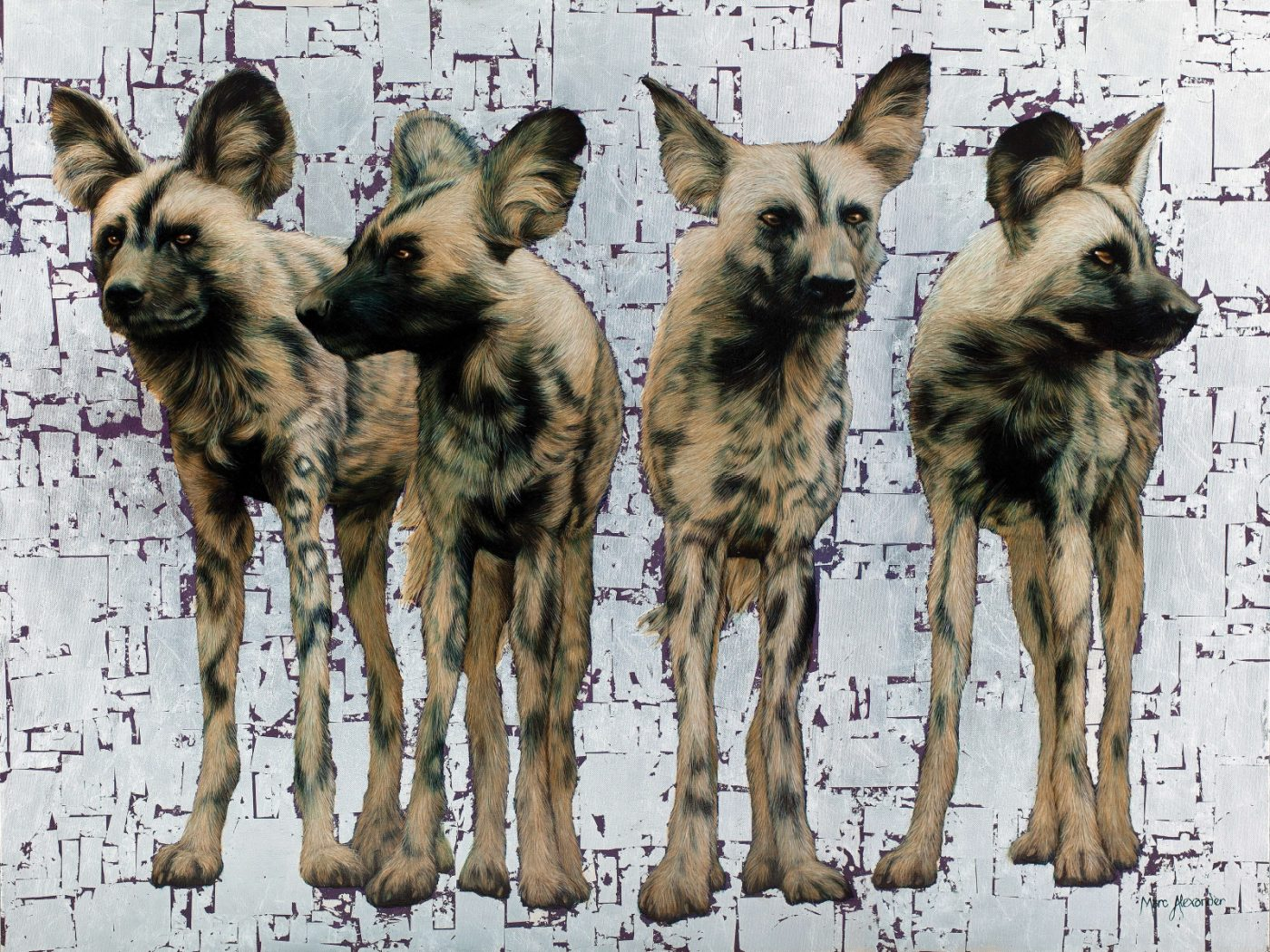##### Wild Dogs Pack of 4
Currency conversion from USD to: [convert number=3650.00 from="usd" to="zar"] ZAR | [convert number=3650.00 from="usd" to="gbp"] GBP | [convert number=3650.00 from="usd" to="eur"] EUR     Medium: Oil and Silver Leaf on Canvas Size: 90cm by 120cm SOLD
\$ 3,650.00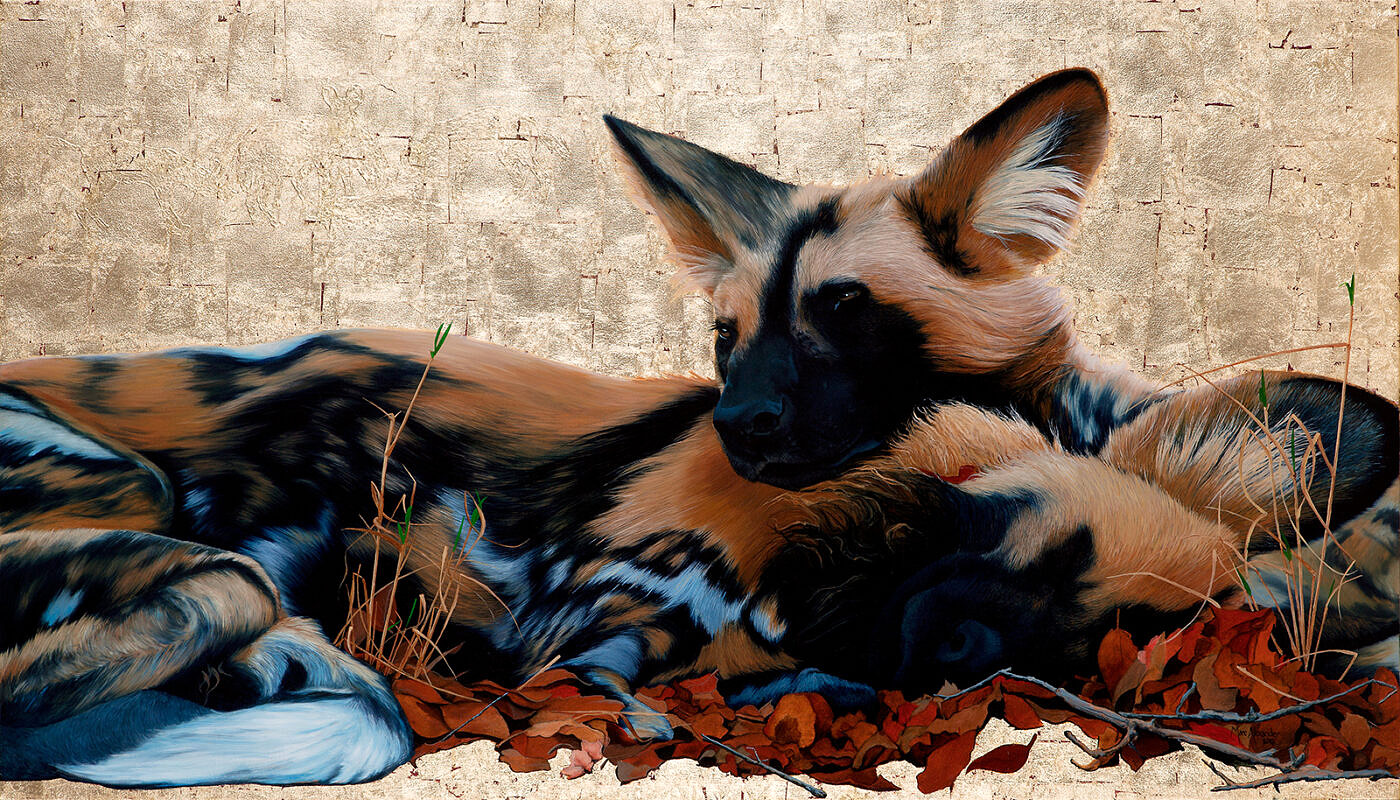##### Wild Dogs I
Currency conversion from USD to: [convert number=3650.00 from="usd" to="zar"] ZAR | [convert number=3650.00 from="usd" to="gbp"] GBP | [convert number=3650.00 from="usd" to="eur"] EUR     Medium: Oil and Gold Leaf on Canvas Size: 80cm by 140cm SOLD
\$ 3,650.00##### Wild Dogs at Dawn
Currency conversion from USD to: [convert number=3650.00 from="usd" to="zar"] ZAR | [convert number=3650.00 from="usd" to="gbp"] GBP | [convert number=3650.00 from="usd" to="eur"] EUR     Medium: Oil and Gold Leaf on Canvas Size: 80cm by 140cm SOLD
\$ 3,650.00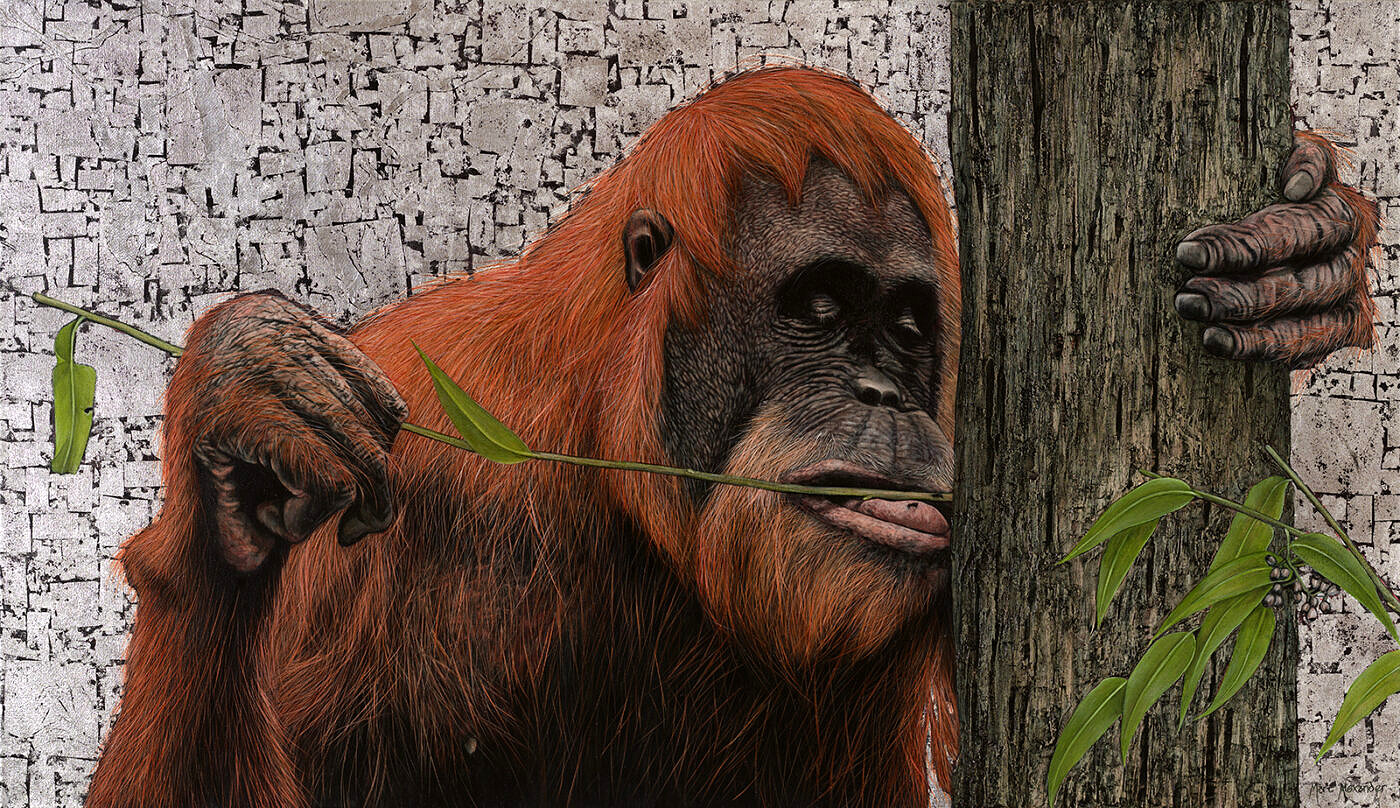##### Sumatran Orangutan
Currency conversion from USD to: [convert number=3650.00 from="usd" to="zar"] ZAR | [convert number=3650.00 from="usd" to="gbp"] GBP | [convert number=3650.00 from="usd" to="eur"] EUR     Medium: Oil and Silver Leaf on Canvas Size: 80cm by 140cm SOLD
\$ 3,650.00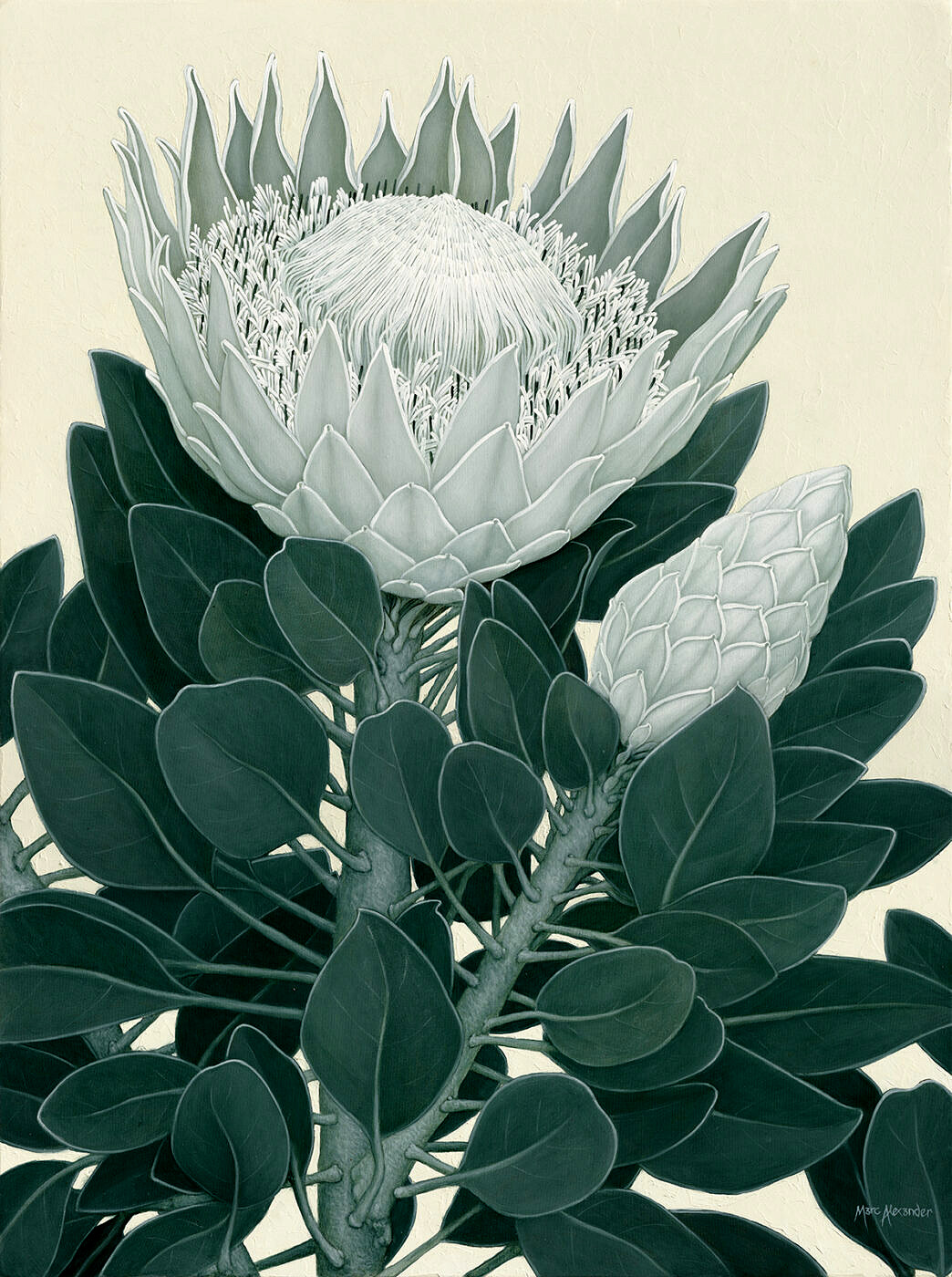##### King Protea II
Currency conversion from USD to: [convert number=3650.00 from="usd" to="zar"] ZAR | [convert number=3650.00 from="usd" to="gbp"] GBP | [convert number=3650.00 from="usd" to="eur"] EUR     Medium: Oil on Canvas Size: 1200cm by 90cm SOLD
\$ 3,650.00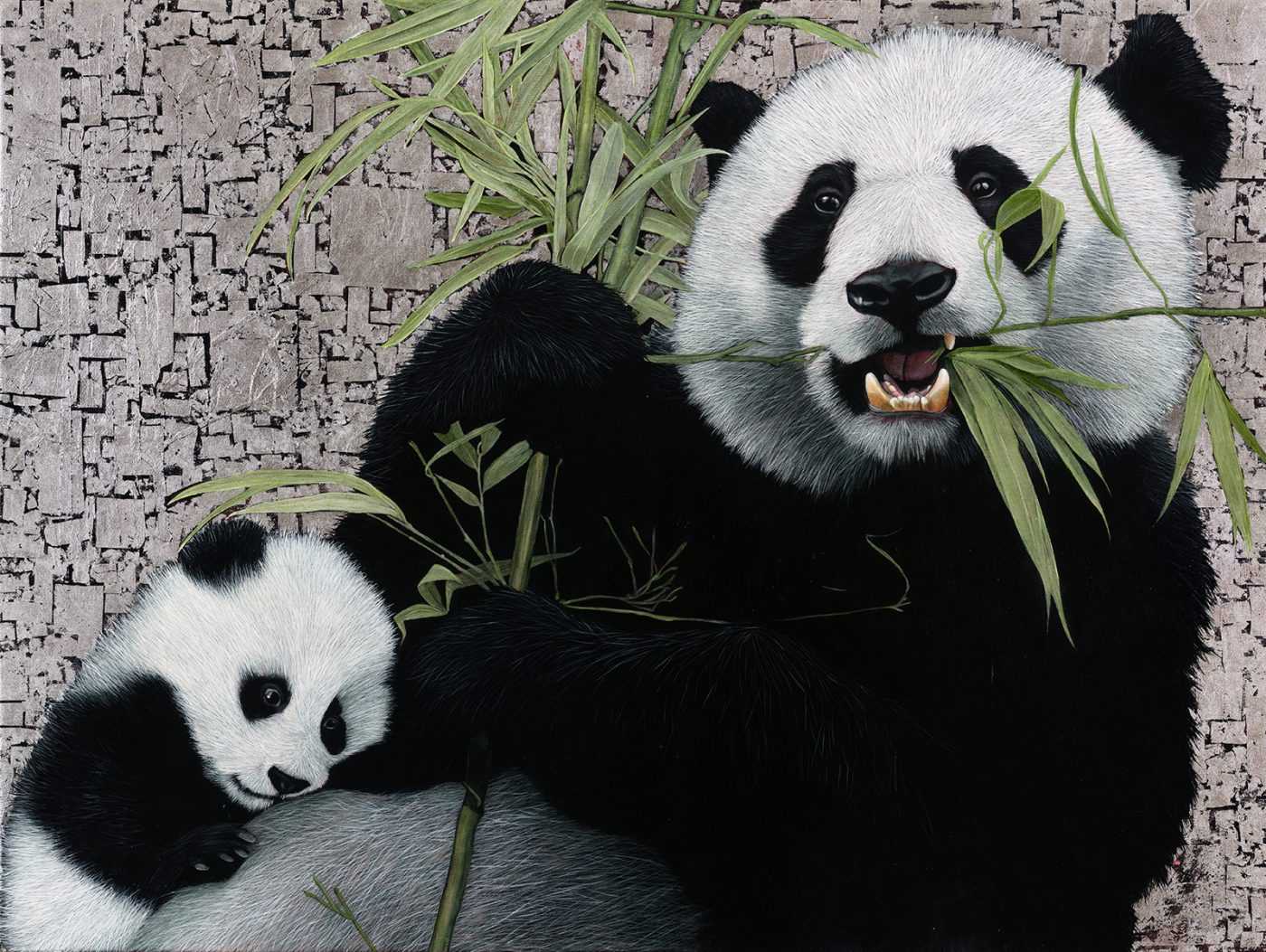##### Giant Pandas
Currency conversion from USD to: [convert number=3700.00 from="usd" to="zar"] ZAR | [convert number=3700.00 from="usd" to="gbp"] GBP | [convert number=3700.00 from="usd" to="eur"] EUR   Medium: Oil and Silver Leaf on Canvas Size: 90cm by 120cm SOLD
\$ 3,700.00##### Bateleur Eagle II
Currency conversion from USD to: [convert number=3850.00 from="usd" to="zar"] ZAR | [convert number=3850.00 from="usd" to="gbp"] GBP | [convert number=3850.00 from="usd" to="eur"] EUR     Medium: Oil and Gold Leaf on Canvas Size: 120cm by 90cm SOLD
\$ 3,850.00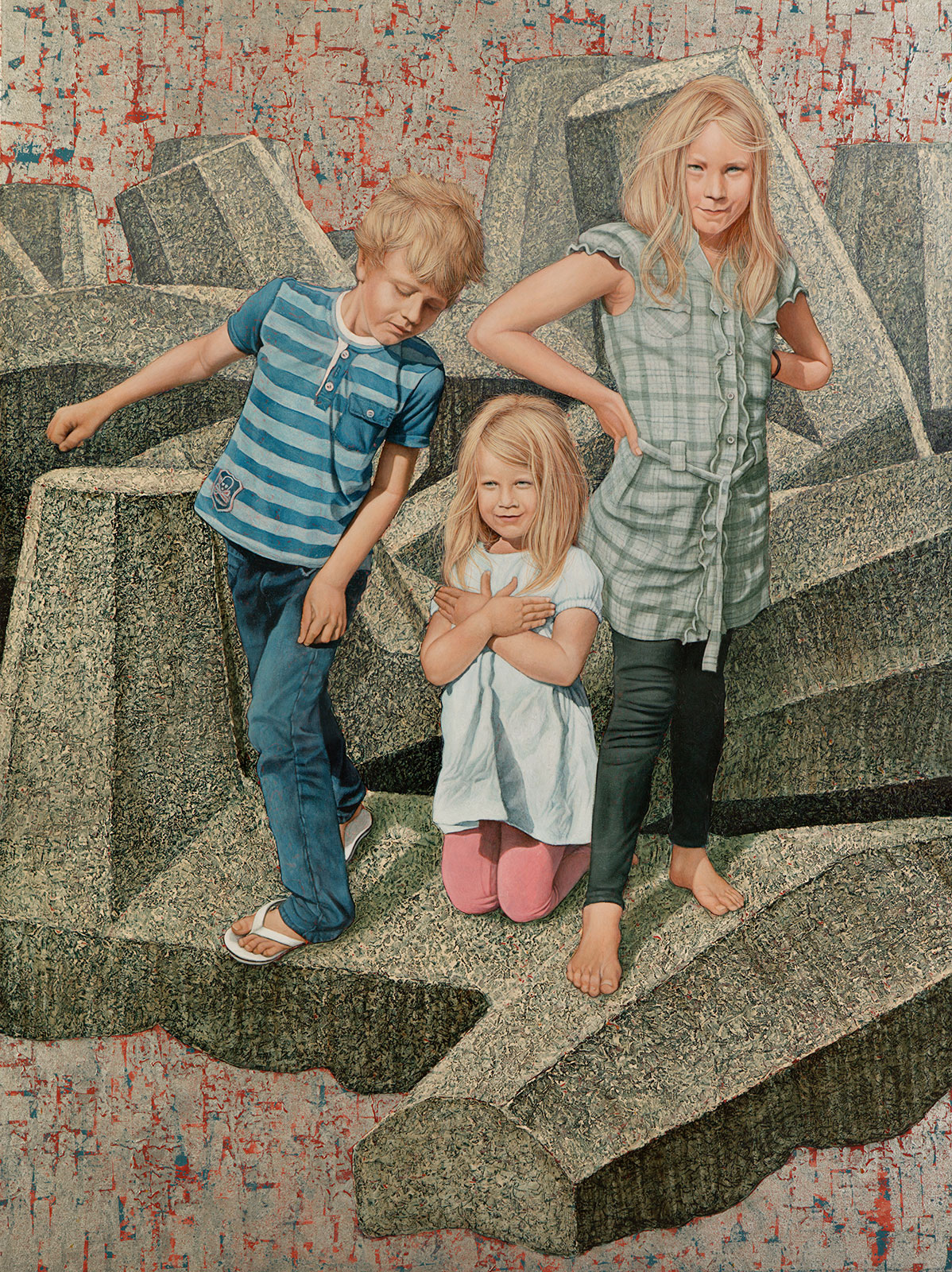##### Child’s Play
Currency conversion from USD to: [convert number=4500.00 from="usd" to="zar"] ZAR | [convert number=4500.00 from="usd" to="gbp"] GBP | [convert number=4500.00 from="usd" to="eur"] EUR     Medium: Oil and Silver Leaf on Canvas Size: 120cm by 90cm
\$ 4,500.00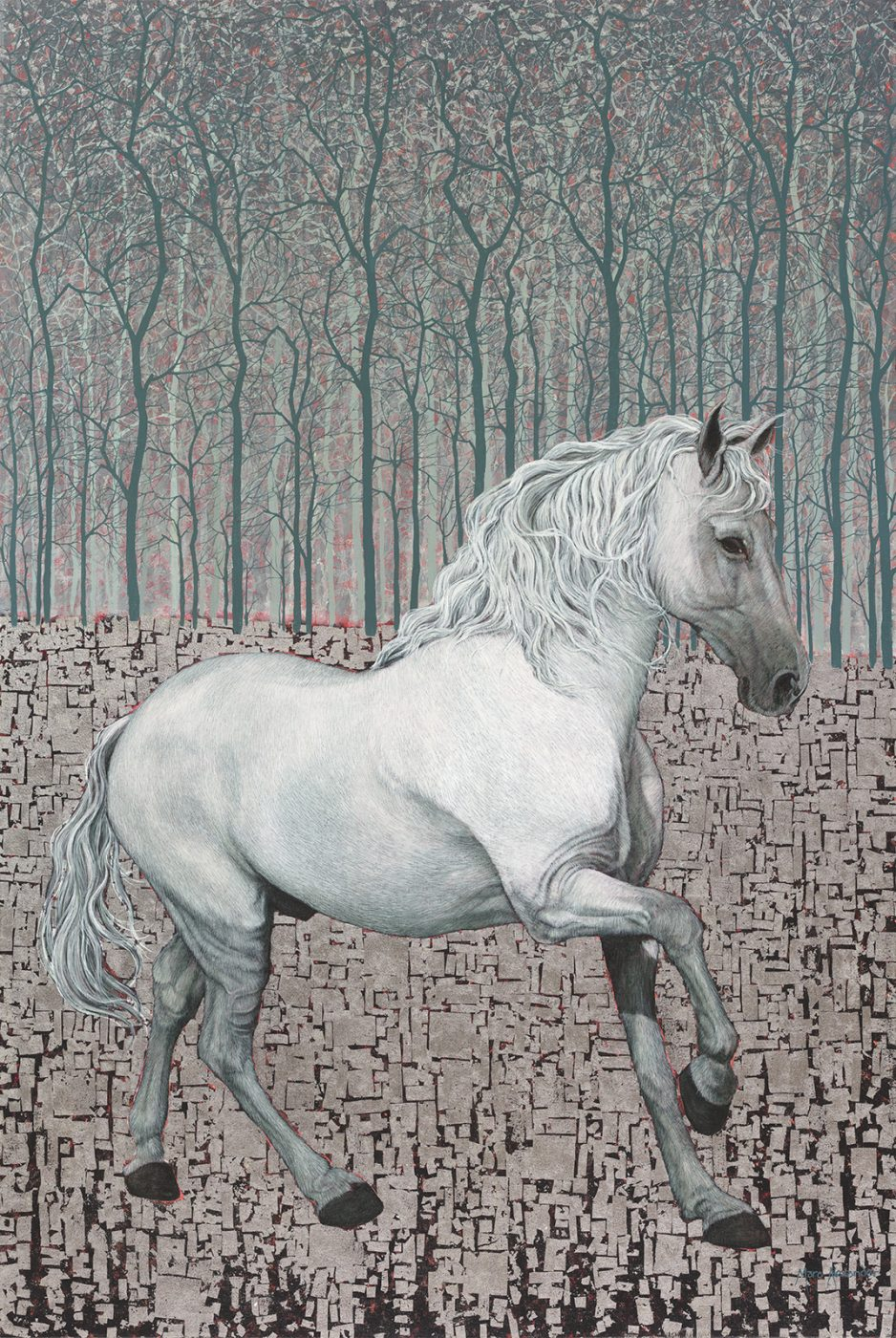Currency conversion from USD to: [convert number=4600.00 from="usd" to="zar"] ZAR | [convert number=4600.00 from="usd" to="gbp"] GBP | [convert number=4600.00 from="usd" to="eur"] EUR   Medium: Oil and Silver Leaf on Canvas Size: 100cm by 150cm SOLD
\$ 4,600.00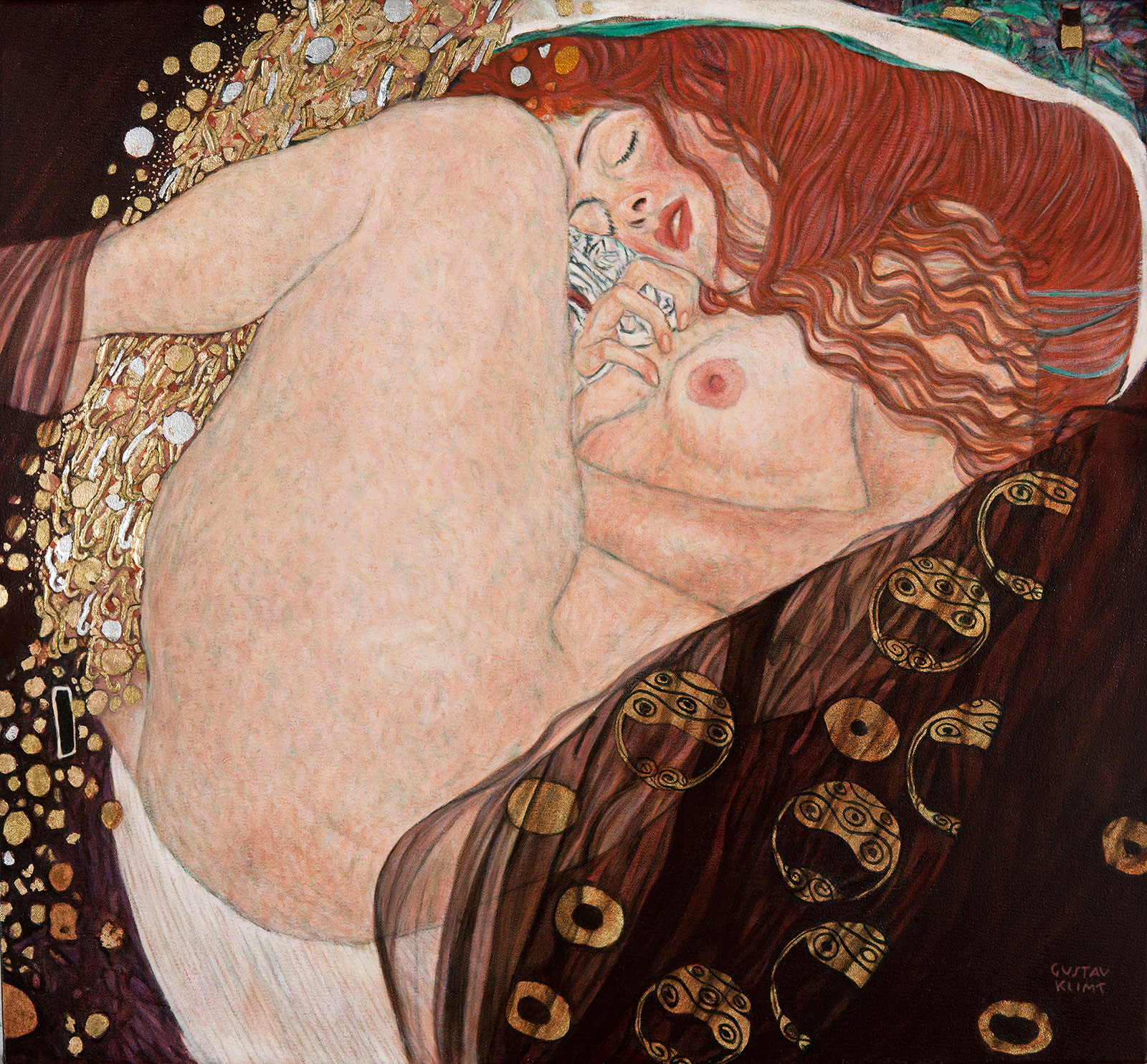##### Danaë After Klimt
Currency conversion from USD to: [convert number=4850.00 from="usd" to="zar"] ZAR | [convert number=4850.00 from="usd" to="gbp"] GBP | [convert number=4850.00 from="usd" to="eur"] EUR     Medium: Oil and Gold Leaf on Canvas Size: 77cm by 83cm Click here to order a Reproduction
\$ 4,850.00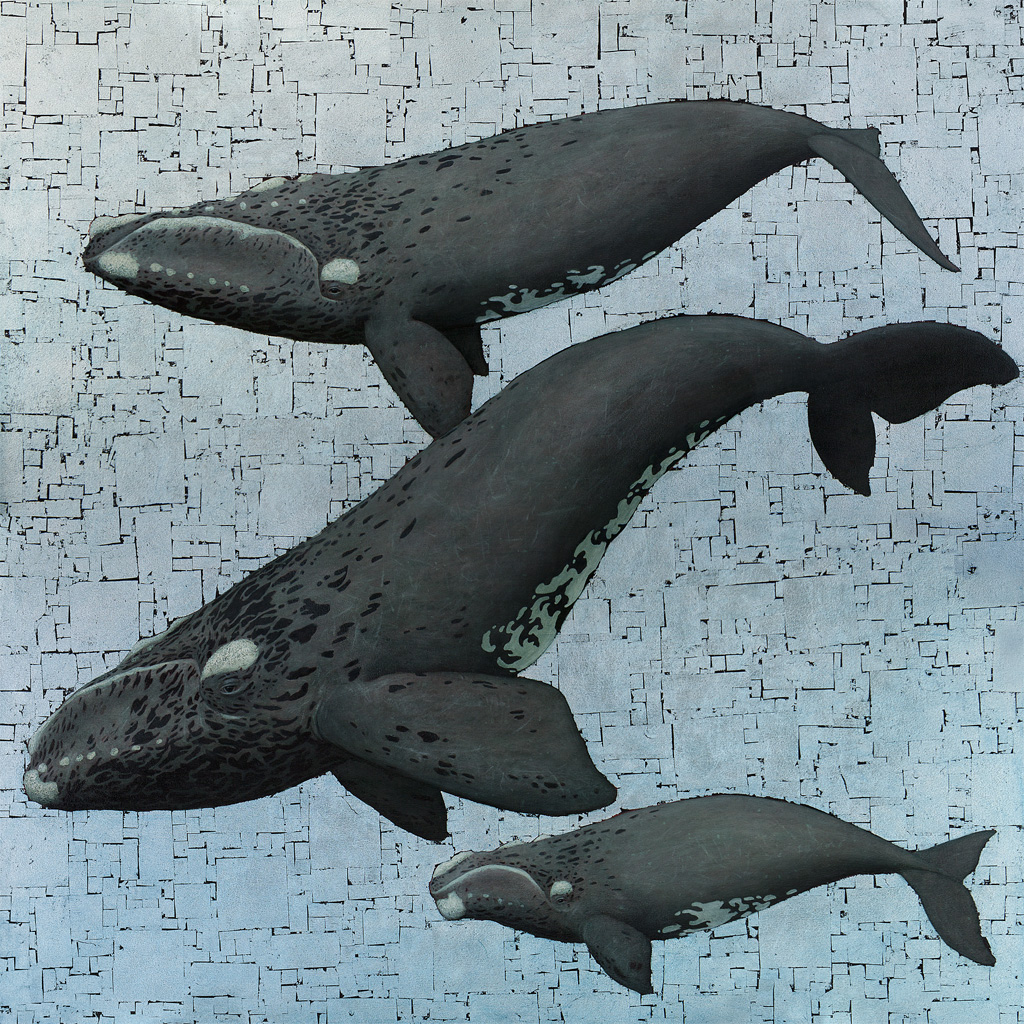##### Whale Season
Currency conversion from USD to: [convert number=7250.00 from="usd" to="zar"] ZAR | [convert number=7250.00 from="usd" to="gbp"] GBP | [convert number=7250.00 from="usd" to="eur"] EUR     Medium: Oil and Silver leaf on Canvas Size: 138cm by 138cm SOLD
\$ 7,250.00##### The Kiss after Klimt
Currency conversion from USD to: [convert number=8150.00 from="usd" to="zar"] ZAR | [convert number=8150.00 from="usd" to="gbp"] GBP | [convert number=8150.00 from="usd" to="eur"] EUR     Medium: Oil and Gold Leaf on Canvas Size: 180cm by 180cm Click here to order a Reproduction
\$ 8,150.00##### Snow Leopard II
Currency conversion from USD to: [convert number=10050.00 from="usd" to="zar"] ZAR | [convert number=10050.00 from="usd" to="gbp"] GBP | [convert number=10050.00 from="usd" to="eur"] EUR     Medium: Oil on Canvas Size: 120cm by 200cm SOLD
\$ 10,050.00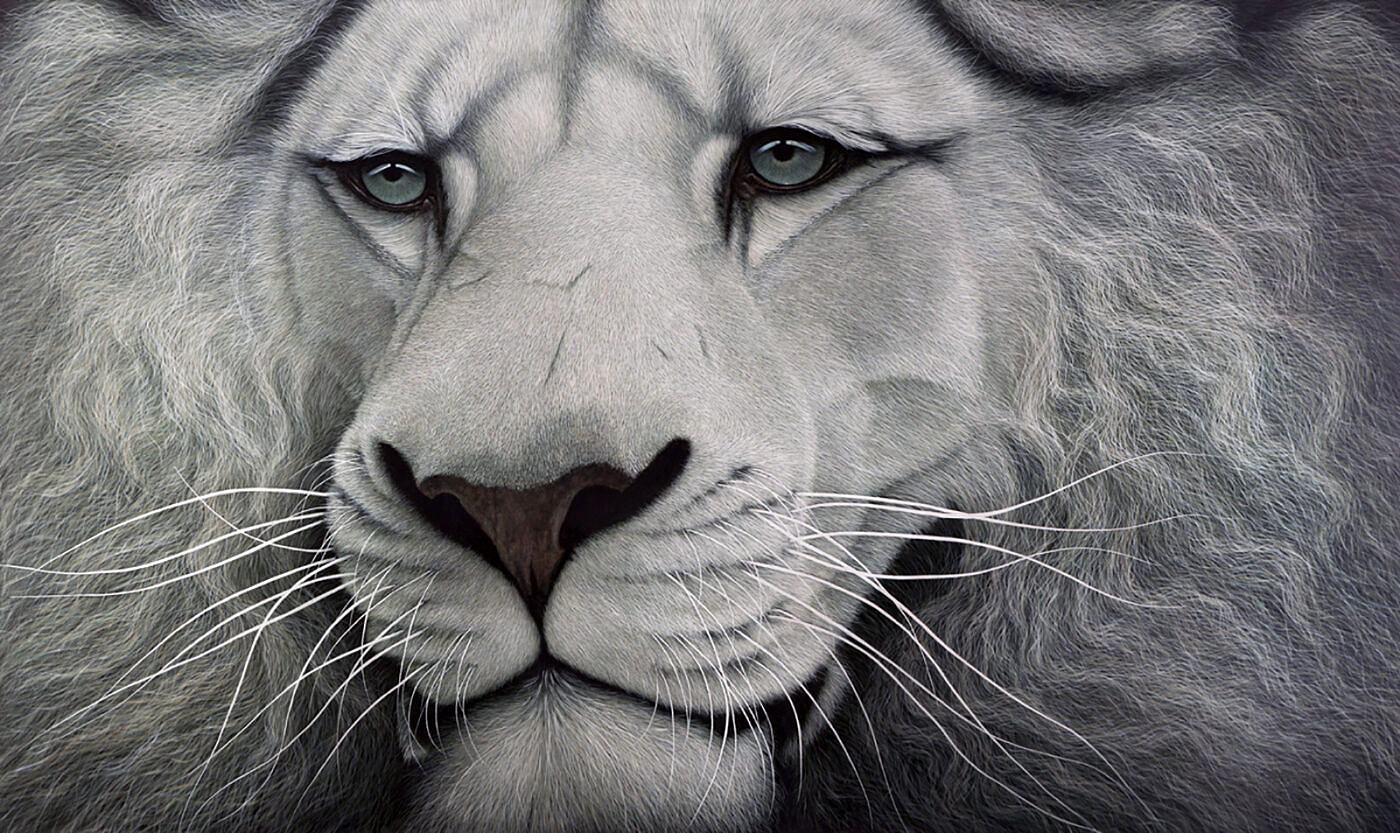##### White Lion
Currency conversion from USD to: [convert number=11000.00 from="usd" to="zar"] ZAR | [convert number=11000.00 from="usd" to="gbp"] GBP | [convert number=11000.00 from="usd" to="eur"] EUR     Medium: Oil on Canvas Size: 120cm by 200cm SOLD
\$ 11,000.00